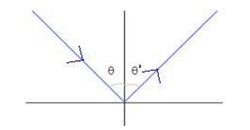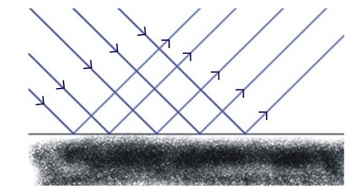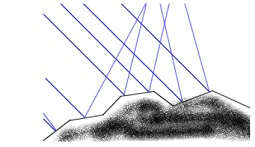# Reflection of light

## What is REFLECTION OF LIGHT

When a beam of light falls on any surface, a part of it is sent back into the same medium from which it is coming. This phenomenon is known as the reflection of light.

• The ray of light which falls on the mirror surface is called the incident ray. The angle of incidence is the angle made by the incident ray with the normal at the point of incidence.
•  The ray of light which is sent back by the mirror is called the reflected ray. The angle of reflection is the angle made by the reflected ray with the normal at the point of incidence.
• The normal is a line at right angle to the mirror surface at the point of incidence.

To score More in your class 8 refer NCERT solutions for class 8 .

### LAWS OF REFLECTION:

(i) Incident ray, normal ray and the reflected ray, all lie in the same plane.

(ii) The angle of reflection is always equal to the angle of incidence.

∠i = ∠r [∠I =  angle of reflection, ∠r = angle of incidence]

### Law of reflection:

θ′ = θ where θ is the angle of incidence and θ' is the reflected angle from the normal.Example1. What happens when a ray of light falls normally (or perpendicularly) on the surface of a mirror ?

Sol. A ray of light which is incident normally on a mirror, is reflected back along the same path because the angle of incidence as well as angle of reflection for such a ray of light are zero.

### TYPES OF REFLECTION:

• Regular reflection or Specular reflection : When a parallel beam of light is incident on a plane highly polished surface, the reflected beam will also be parallel and hence the whole light falling on the surface is reflected in a definite direction. Such a reflection is called regular reflection. All the reflected rays are in line with each other.Such a surface is called a reflector, like a plane mirror, a polished metal surface.

• Irregular reflection or diffused reflection: When a parallel beam of light is incident on rough surface or irregular surface, the rays get reflected in all directions and the reflected light spreads over a wide area. The small bumps and irregularities on a rough surface will cause each of the light rays to reflect in different directions, all following the law of reflection of course. This reflection is known as irregular or diffused reflection.